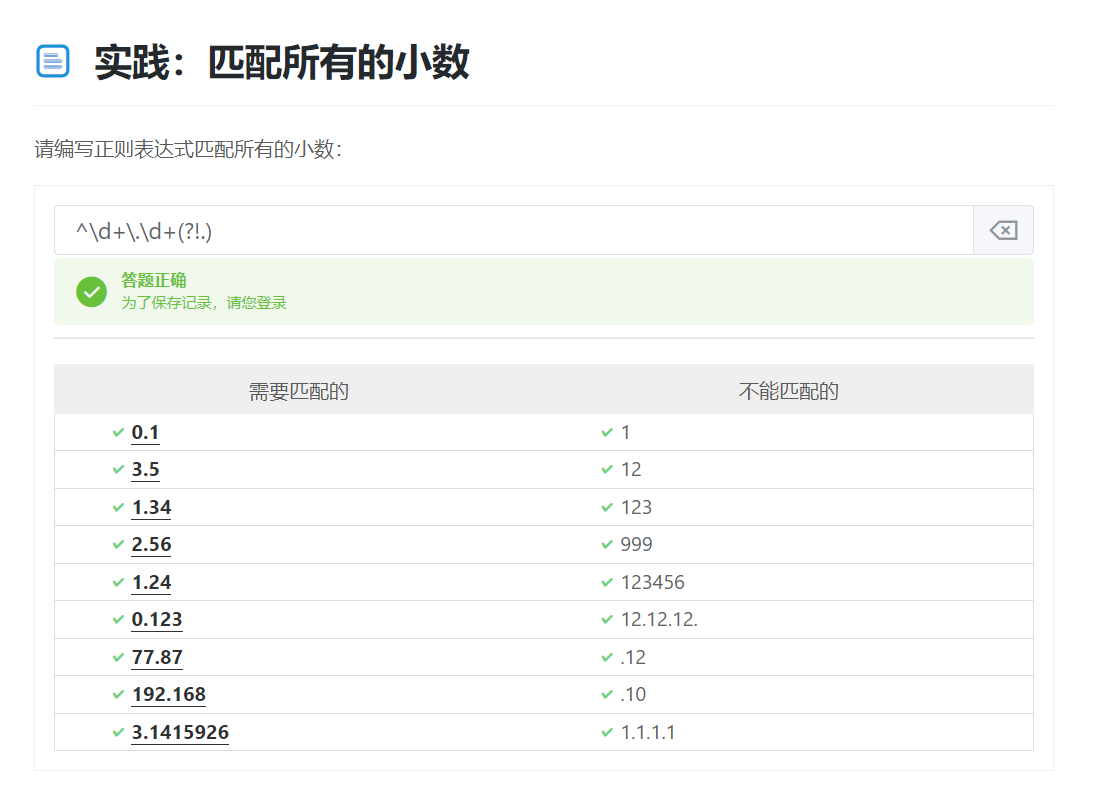# python正则表达式进阶

## 1、分组

### 1.分组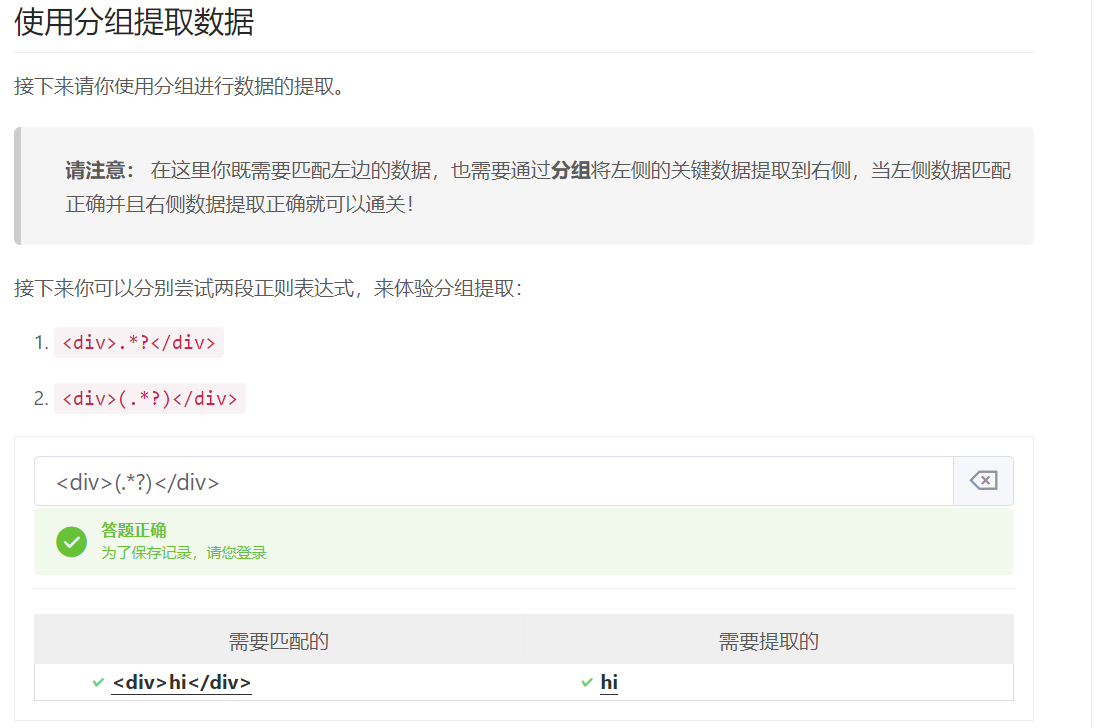### 2.提取p标签中的数据

<p>(.*)</p>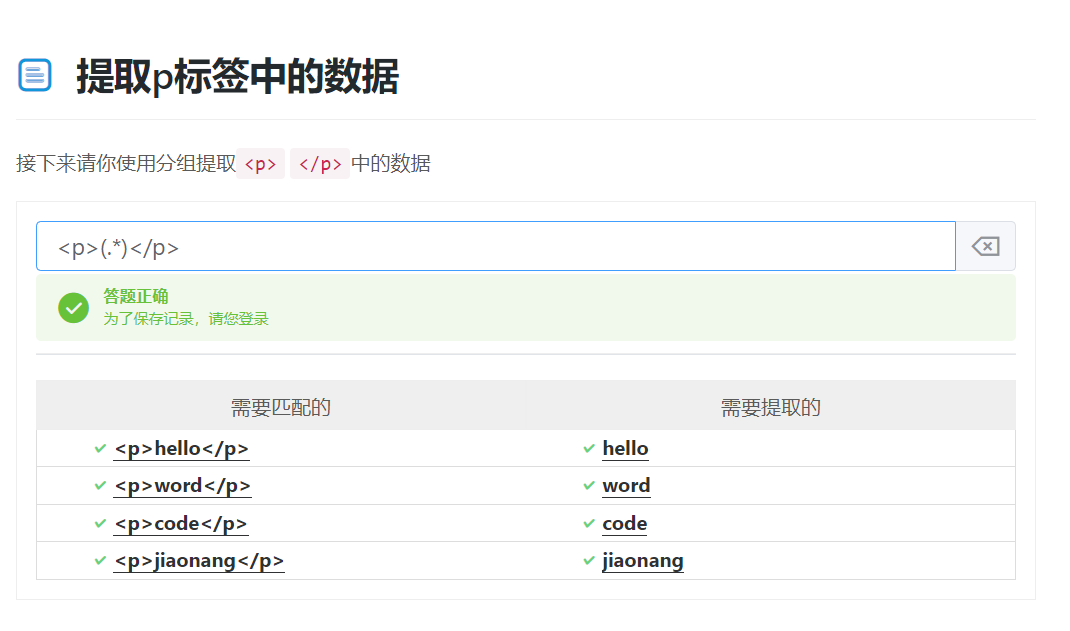### 3.提取学号

(\d{4})[-\s]?(\d{4})[\s-]?(\d{2})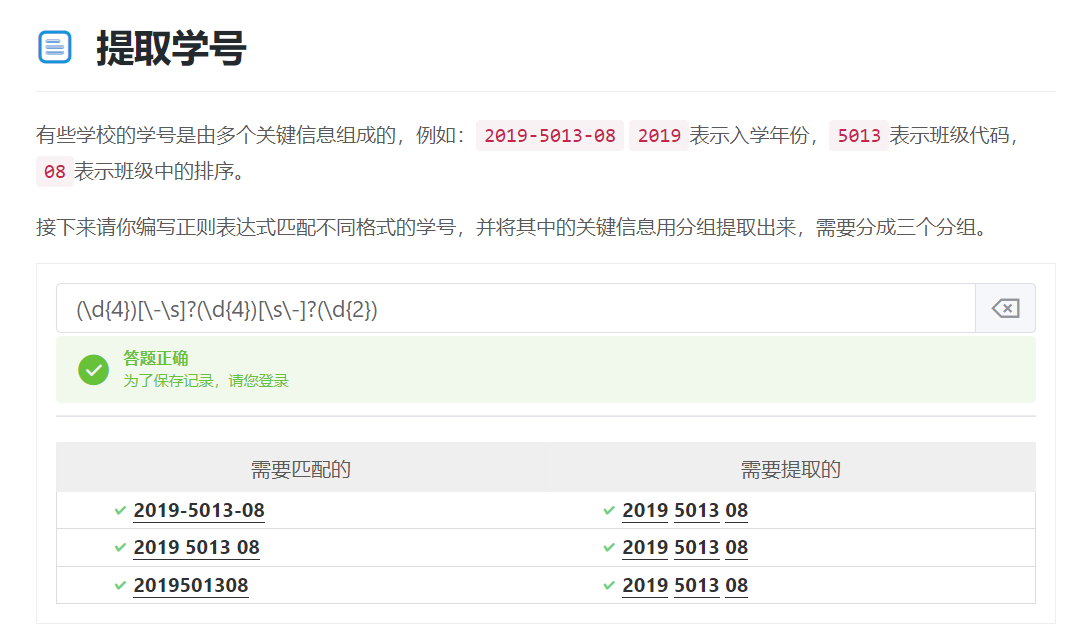### 4.提取年月日

(\d{4})-/-/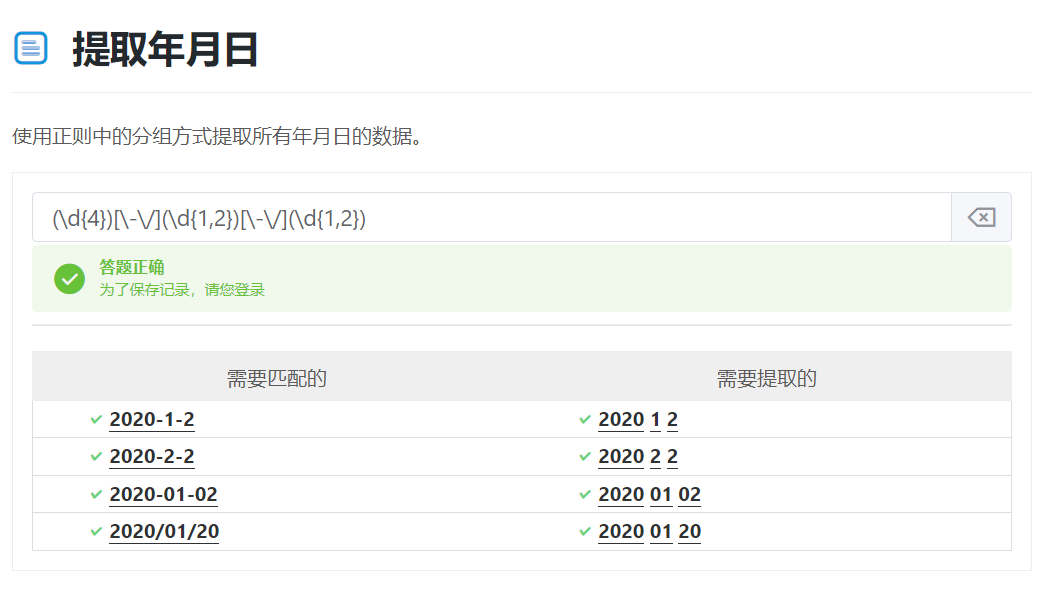### 5.或者条件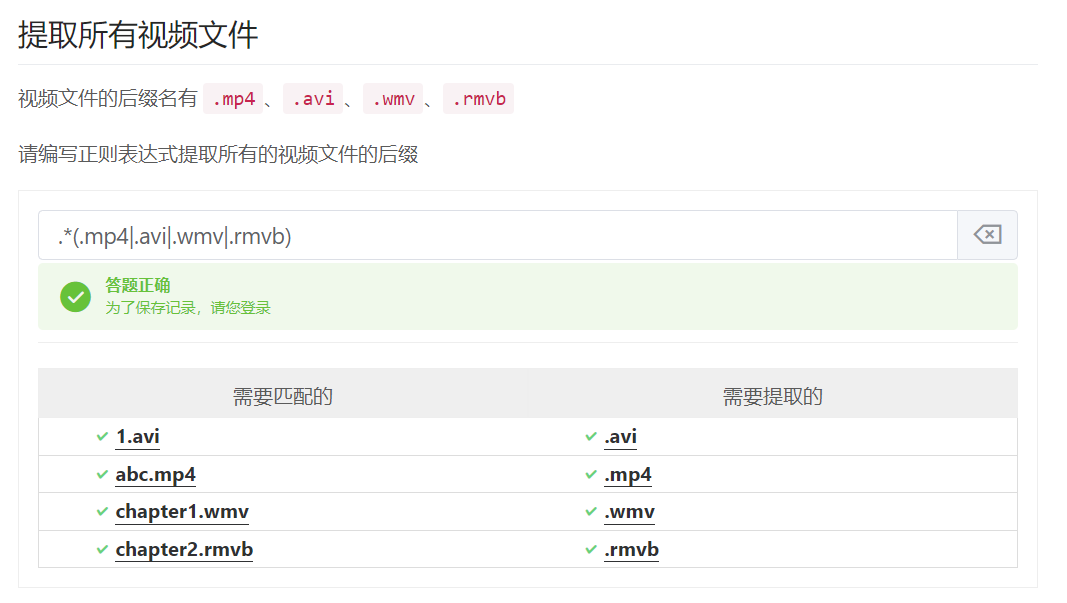### 6.非捕获分组

(?:\d*-|tel:)(\d*)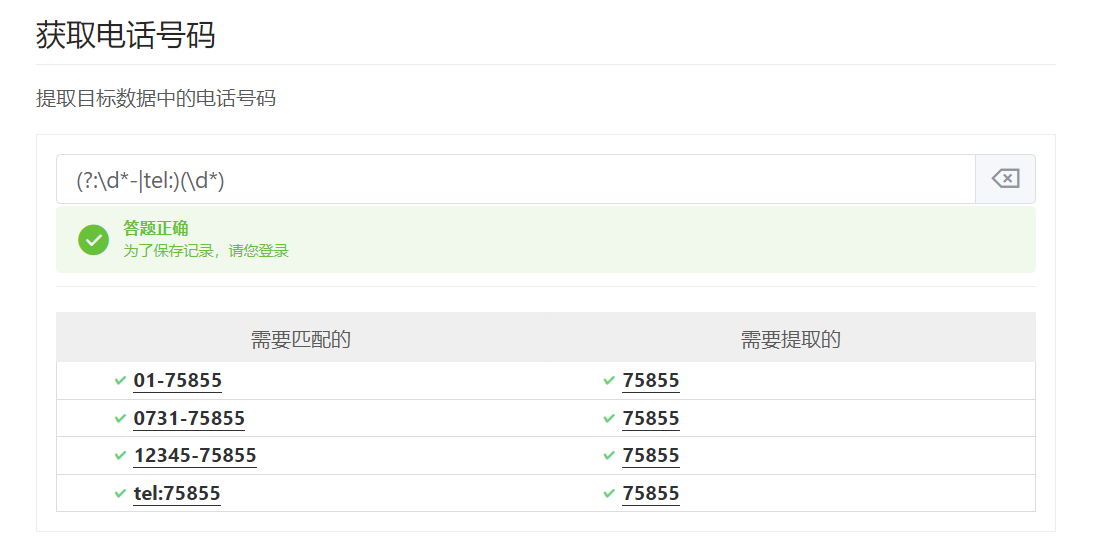### 7.分组使用技巧

(\d{4})[-\s./]?(\d{1,2})[-\s./]?(\d{1,2})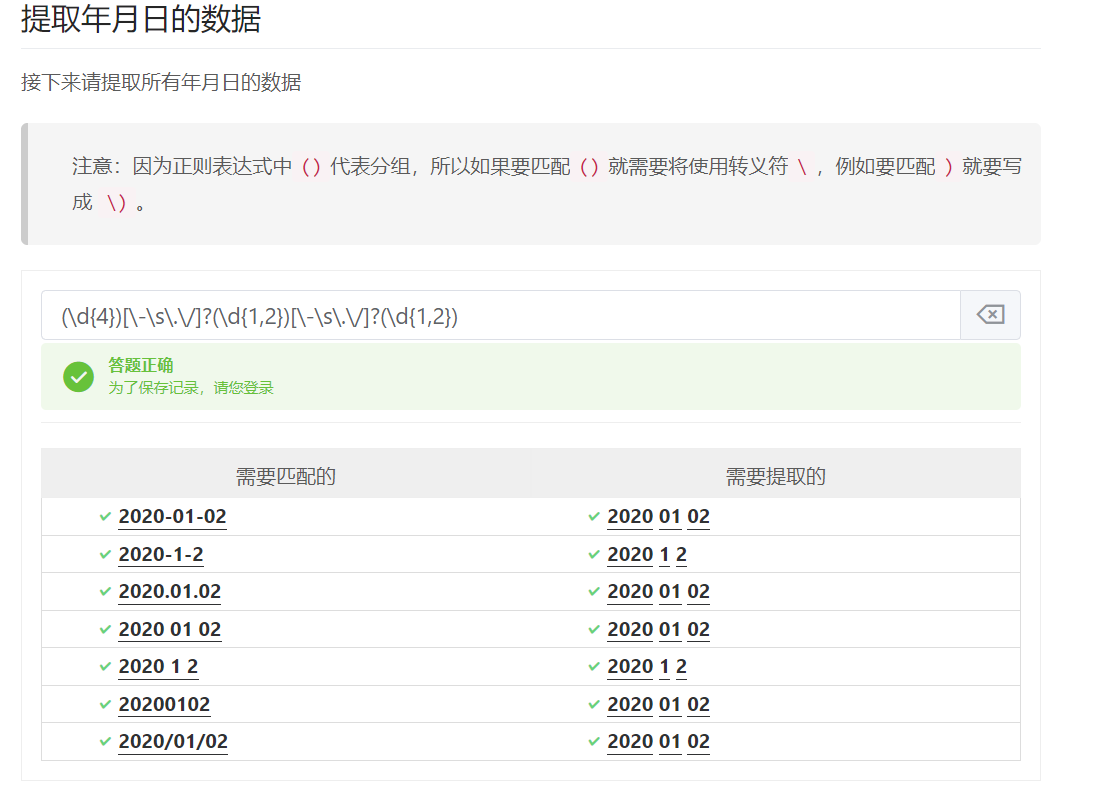### 8.提取所有电话号码

(\d{3})[).-]?(\d{3})[.-]?(\d{4})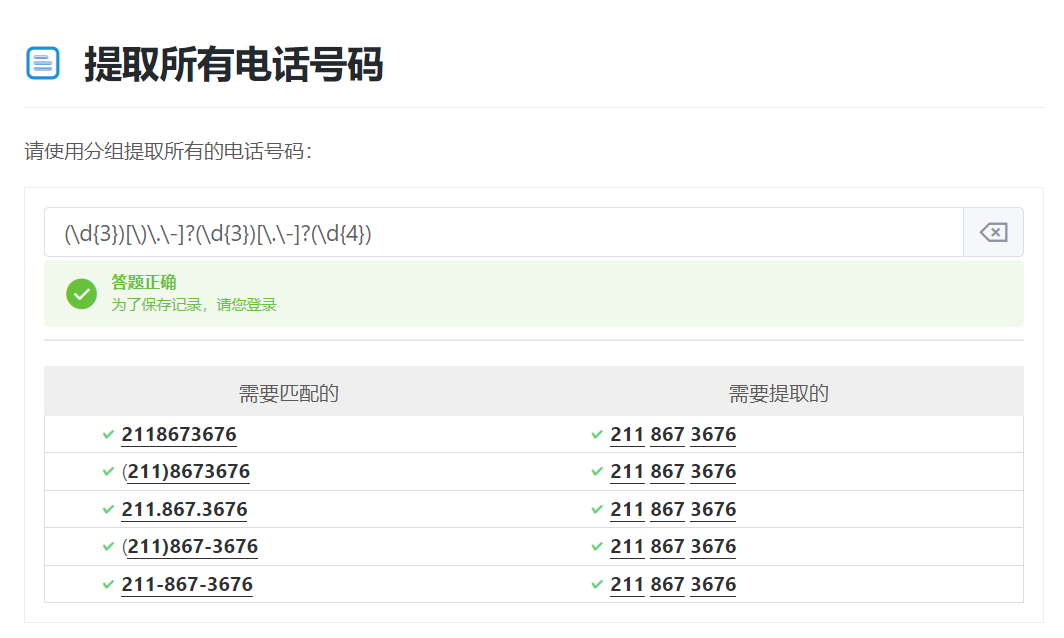### 9.分组的回溯引用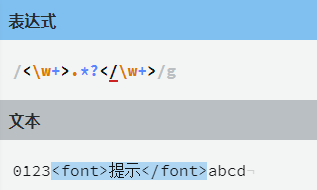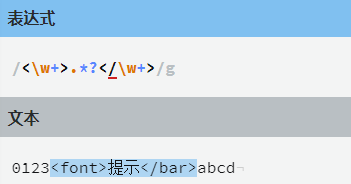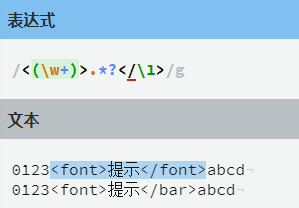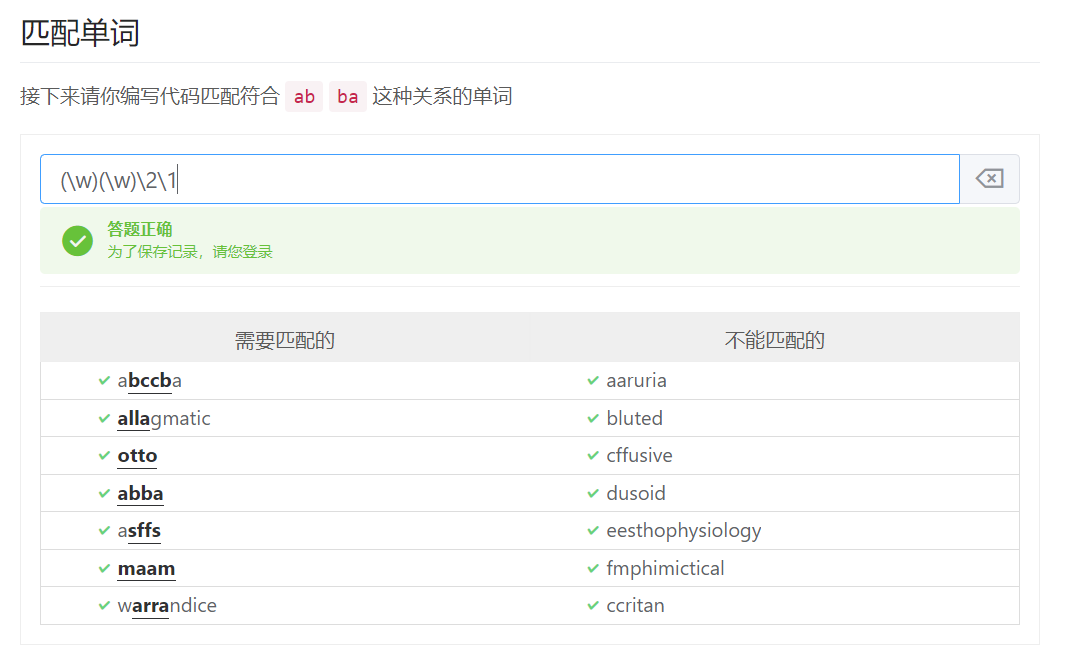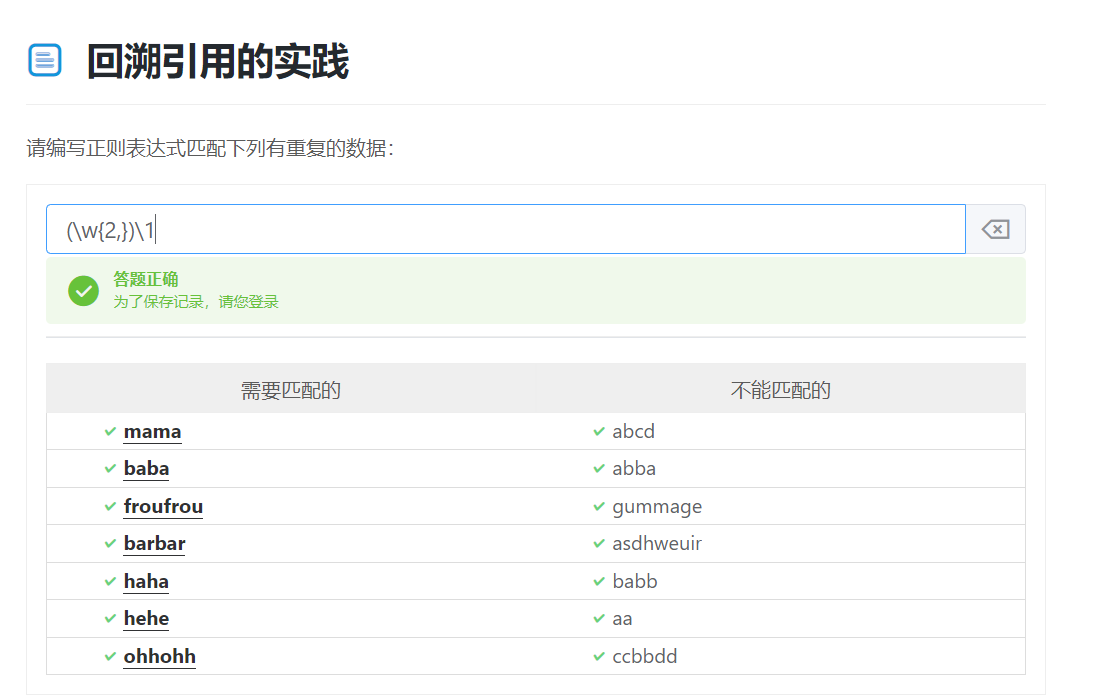## 2、先行断言

1. 正向先行断言
2. 反向先行断言
3. 正向后行断言
4. 反向后行断言

### 1.正向先行断言

(?=.?[a-z])(?=.[A-Z])(?=.*\d).{8,}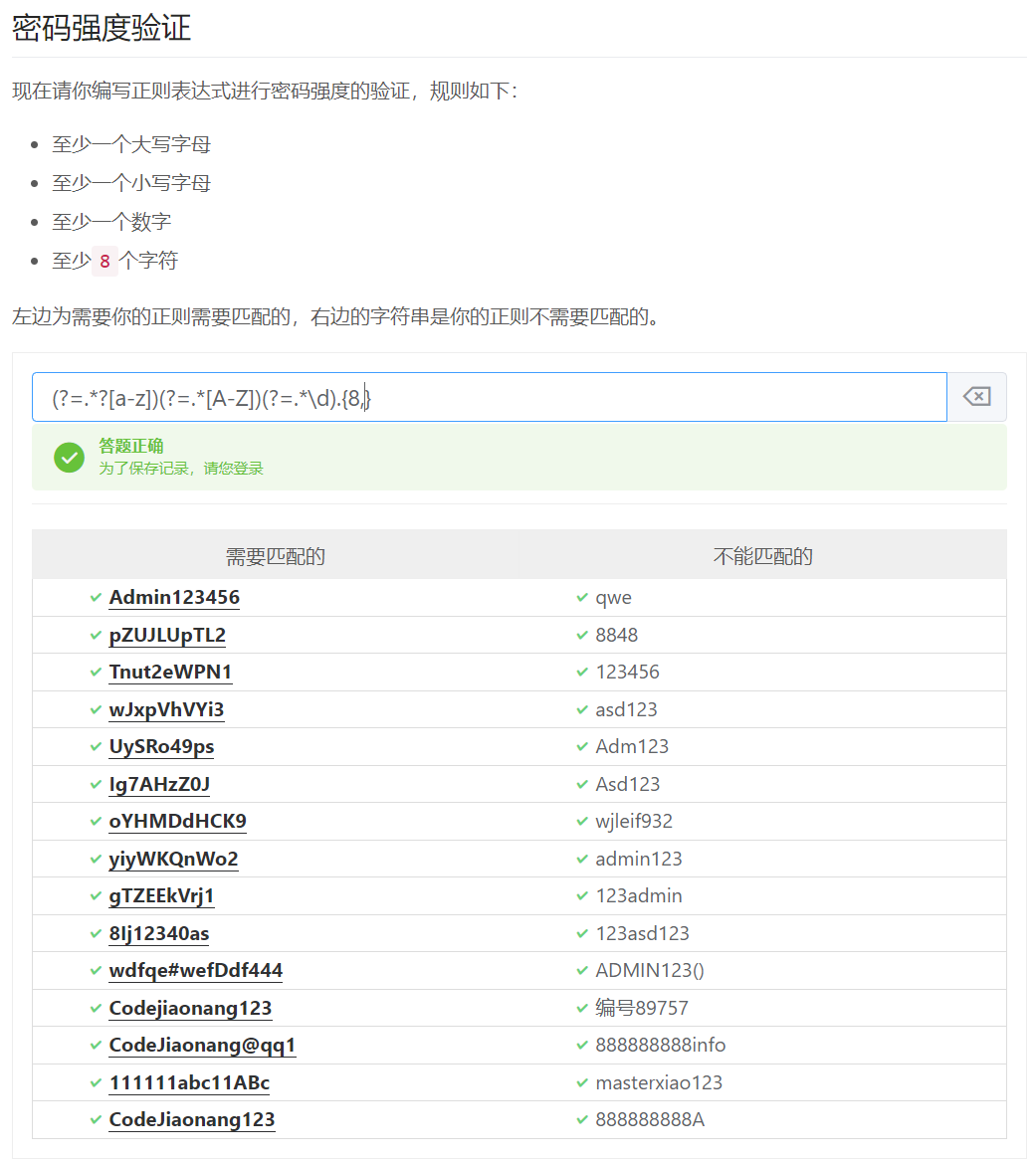### 2.反向先行断言

.+@(?!qq).+.com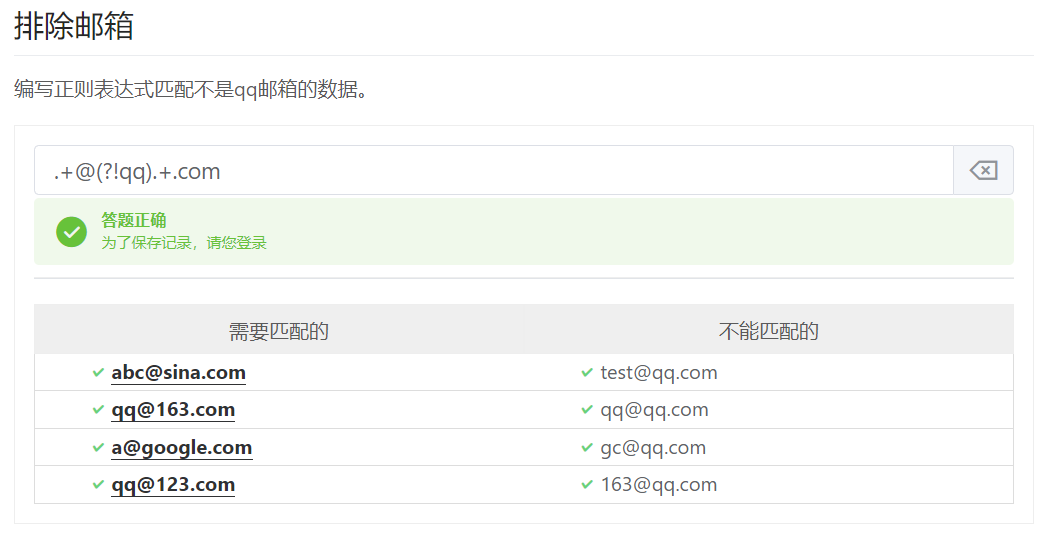<((?!p).+)>.*</\1>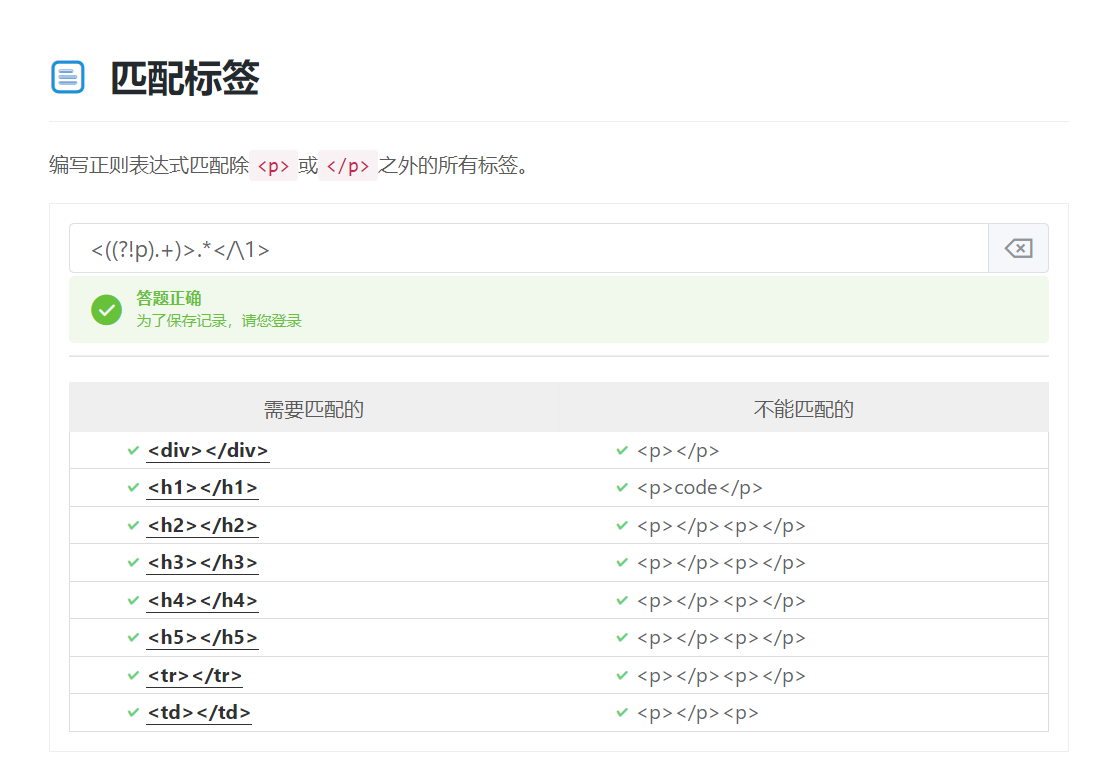## 3、后行断言

### 1.正向后行断言

(王)(?<=王).+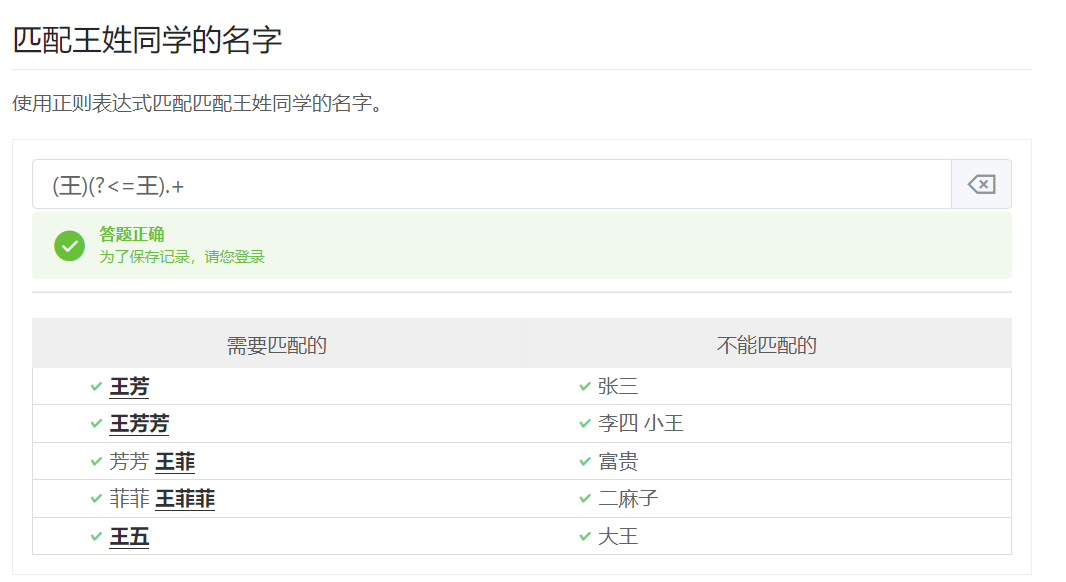### 2.反向后行断言

^\$.*(?<!\$)\$\$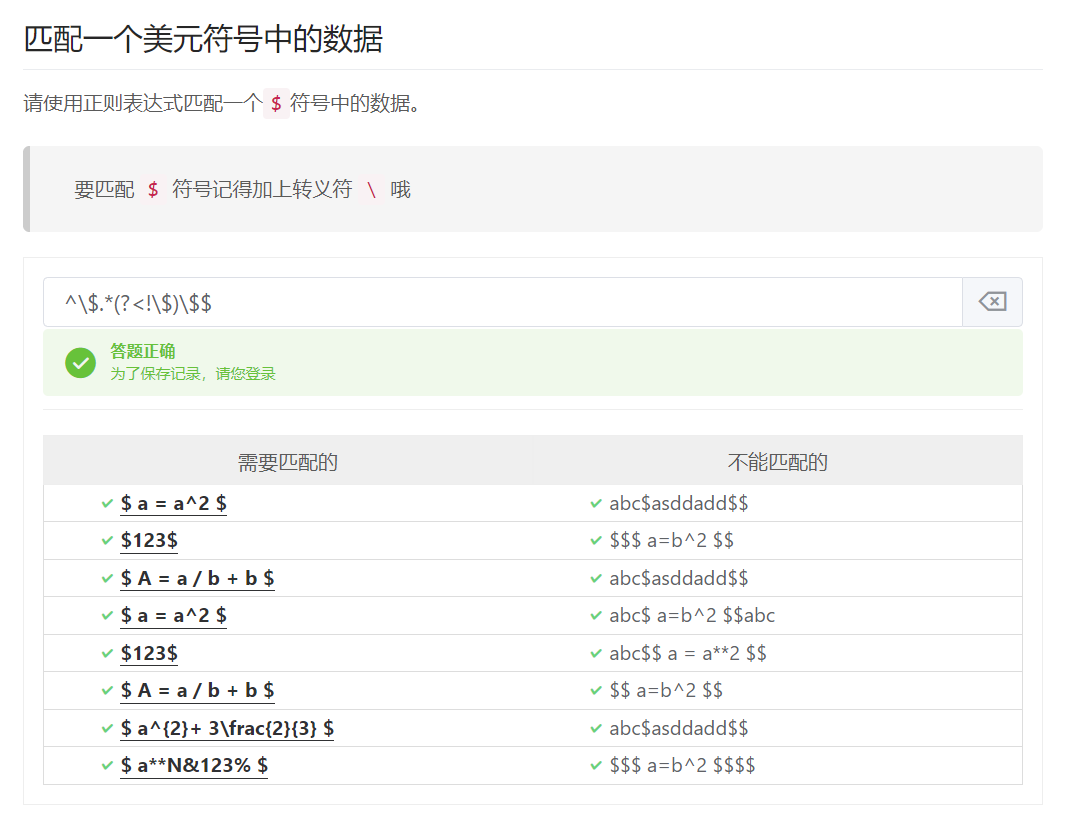(?<!\\$)\\$\\$[^\\$].*\\$\\$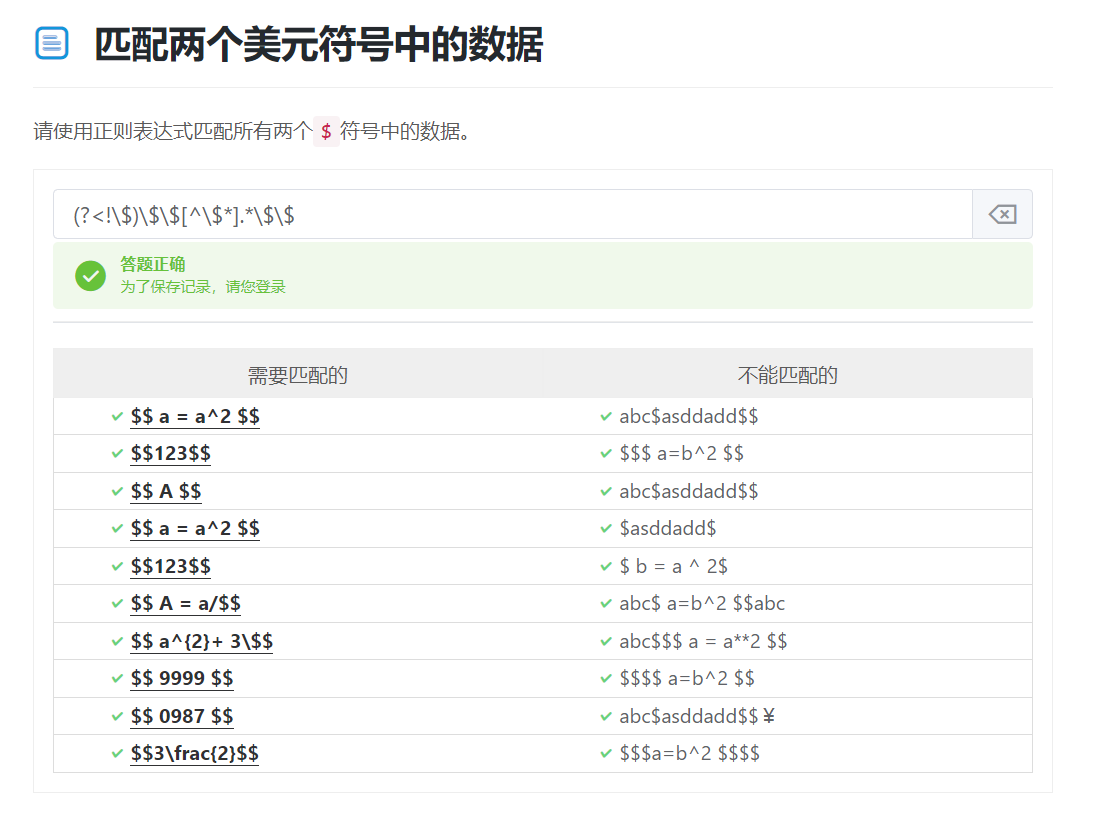## 4、总结与实践

### 1.提取所有人的生日

``````.*[\s]?\d{4}[\S](\d{1,2})[\S](\d{1,2}).*
``````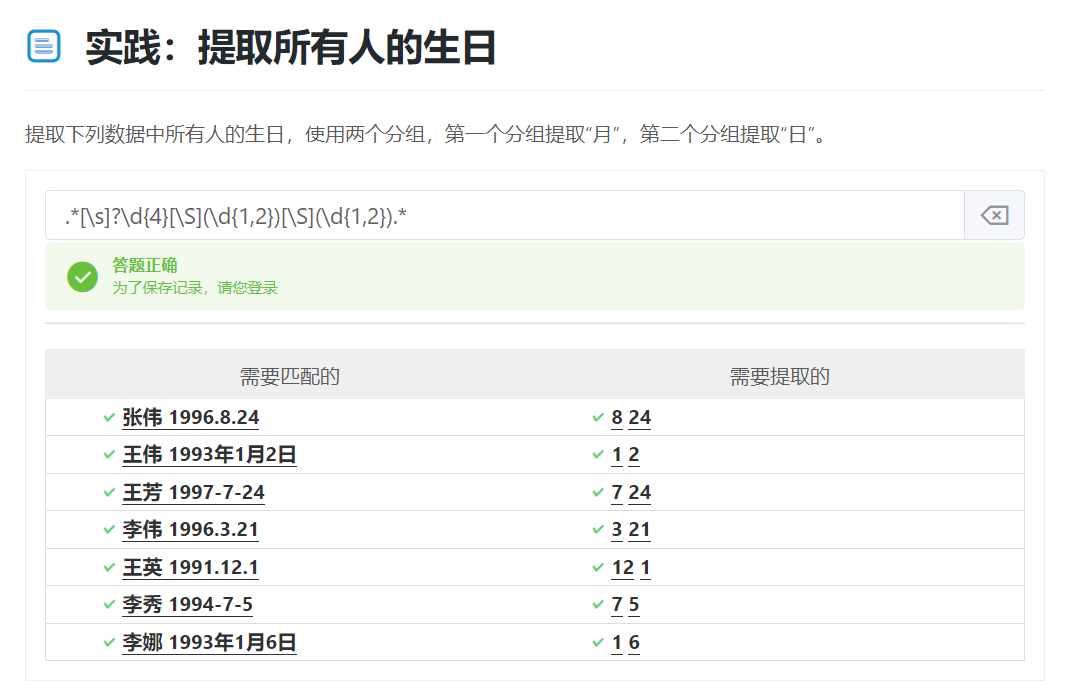### 2.匹配所有的小数

``````^\d+\.\d+(?!.)
``````